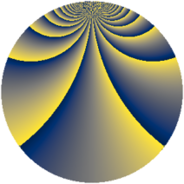# Properties

 Label 1840.2.uLevel $1840$ Weight $2$ Character orbit 1840.u Rep. character $\chi_{1840}(91,\cdot)$ Character field $\Q(\zeta_{4})$ Dimension $384$ Sturm bound $576$

# Related objects

## Defining parameters

 Level: $$N$$ $$=$$ $$1840 = 2^{4} \cdot 5 \cdot 23$$ Weight: $$k$$ $$=$$ $$2$$ Character orbit: $$[\chi]$$ $$=$$ 1840.u (of order $$4$$ and degree $$2$$) Character conductor: $$\operatorname{cond}(\chi)$$ $$=$$ $$368$$ Character field: $$\Q(i)$$ Sturm bound: $$576$$

## Dimensions

The following table gives the dimensions of various subspaces of $$M_{2}(1840, [\chi])$$.

Total New Old
Modular forms 584 384 200
Cusp forms 568 384 184
Eisenstein series 16 0 16

## Trace form

 $$384q - 8q^{4} + 12q^{6} + O(q^{10})$$ $$384q - 8q^{4} + 12q^{6} + 12q^{12} + 32q^{16} - 40q^{18} - 16q^{23} - 8q^{24} - 24q^{27} + 32q^{29} - 20q^{36} + 48q^{39} + 4q^{46} - 80q^{48} - 384q^{49} + 8q^{50} + 64q^{52} - 32q^{54} + 76q^{58} - 24q^{59} - 60q^{62} - 44q^{64} + 16q^{69} + 188q^{72} - 32q^{77} - 92q^{78} - 384q^{81} + 36q^{82} + 32q^{85} + 12q^{92} + 48q^{93} + 72q^{94} - 136q^{96} + 208q^{98} + O(q^{100})$$

## Decomposition of $$S_{2}^{\mathrm{new}}(1840, [\chi])$$ into newform subspaces

The newforms in this space have not yet been added to the LMFDB.

## Decomposition of $$S_{2}^{\mathrm{old}}(1840, [\chi])$$ into lower level spaces

$$S_{2}^{\mathrm{old}}(1840, [\chi]) \cong$$ $$S_{2}^{\mathrm{new}}(368, [\chi])$$$$^{\oplus 2}$$